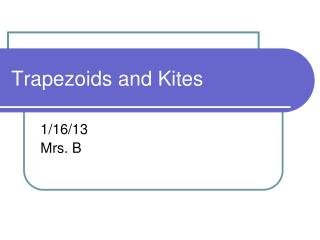DownloadDownload PresentationTrapezoids and Kites

# Trapezoids and Kites

Download Presentation## Trapezoids and Kites

- - - - - - - - - - - - - - - - - - - - - - - - - - - E N D - - - - - - - - - - - - - - - - - - - - - - - - - - -
##### Presentation Transcript

1. Trapezoids and Kites 1/16/13 Mrs. B

2. Objectives: • Use properties of trapezoids. • Use properties of kites.

3. A trapezoid is a quadrilateral with exactly one pair of parallel sides called bases. A trapezoid has two pairs of base angles. Ex. D and C And A and B. The nonparallel sides are the legs of the trapezoid. Using properties of trapezoids

4. If the legs of a trapezoid are congruent, then the trapezoid is an isosceles trapezoid. Using properties of trapezoids

5. If a trapezoid is isosceles, then each pair of base angles is congruent. A ≅ B, C ≅ D Isosceles Trapezoid

6. If a trapezoid is isosceles, then adjacent angles (not bases) are supplementary. <A + <D = 180 <B + <C = 80 Isosceles Trapezoid

7. Given, angle X is 50 Find <R, < P and <Q, Ex. 1: Using properties of Isosceles Trapezoids 50°

8. A trapezoid is isosceles if and only if its diagonals are congruent. ABCD is isosceles if and only if AC ≅ BD. Isosceles Trapezoid

9. The midsegment of a trapezoid is the segment that connects the midpoints of its legs. Midsegment of a trapezoid

10. The midsegment of a trapezoid is parallel to each base and its length is one half the sums of the lengths of the bases. MN║AD, MN║BC MN = ½ (AD + BC) Theorem 6.17: Midsegment of a trapezoid

11. LAYER CAKE A baker is making a cake like the one at the right. The top layer has a diameter of 8 inches and the bottom layer has a diameter of 20 inches. How big should the middle layer be? Ex. 3: Finding Midsegment lengths of trapezoids

12. Use the midsegment theorem for trapezoids. DG = ½(EF + CH)= ½ (8 + 20) = 14” Ex. 3: Finding Midsegment lengths of trapezoids E F D G D C

13. A kite is a quadrilateral that has two pairs of consecutive congruent sides, but opposite sides are not congruent. Using properties of kites

14. Theorem 6.18 If a quadrilateral is a kite, then its diagonals are perpendicular. AC  BD Kite theorems

15. Theorem 6.19 If a quadrilateral is a kite, then exactly one pair of opposite angles is congruent. A ≅ C B not =D Kite theorems

16. WXYZ is a kite so the diagonals are perpendicular. You can use the Pythagorean Theorem to find the side lengths. WX = XY = Ex. 4: Using the diagonals of a kite

17. Ex. 5: Angles of a kite • Find mG and mJ in the diagram. 132° 60°